# How to explain deviations in operational amplifier circuits

#### wouter368

Joined Sep 22, 2022
20
Hey,

I need some help with a couple of circuits that I built. The circuits together are supposed to function as an analog calculator. I realize that analog computers can not be 100% accurate, but I wanted to test how inaccurate an analog calculator really is. So I built the following circuits: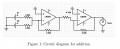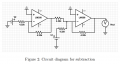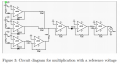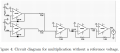I tested the accuracy of Vout in these circuits in the following manner:

- I held V2 at a constant voltage (1,00V)
- I varied V1 from 0 to 11V (with the exception of subtraction where I varied it from -11V to 11V)
- I measured Vout and notated the deviation from the expected answer.

You can see my measurements in this spreadsheet:

There's multiple things that caught my attention here:

- With addition and subtraction, it seems that the higher V1 is, the higher the deviation is. Addition and subtraction are relatively pretty accurate.
- With multiplication it's a little bit more complicated. It seems that until V1 reaches 7V, the deviation increases quite linear. However, after 7V, the measurements stop making sense for me. Firstly, when measuring the voltage, Vout would continuously vary within a range of around 0.5V. This made it hard to measure Vout since in one second, it could change a lot, unexplainably.
- The results for divison hardly allow any logical reasoning for me, since the deviation values seemed completely random. The same behaviour as with the multiplication circuit has been noted; Vout would continously vary within a (although smaller) range of around 0.15V. This made it hard to measure Vout exactly.

So my question is, what could cause this behaviour? For subtraction and addition I feel like it has to do with a positive Common Mode amplification factor. The common mode signal increases if (V1+V2) increases, so that would make sense. It would also explain the linear relationship between V1 and the deviation. Is this a correct analysis?

However, for multiplication and division I have no clue what causes these fluctuations and deviations. It would help a lot if someone could explain this behaviour.

If you have any questions regarding my circuit or the measurements, please let me know. Thanks in advance!

#### ericgibbs

Joined Jan 29, 2010
16,800
hi,
What are the tolerances of the passive components>?
E
These images are copies of your results

Last edited:

#### Audioguru again

Joined Oct 21, 2019
5,418
The lousy old LM358 produces crossover distortion that creates errors near the crossover voltages and it produces a high noise level. It has a wide range of input offset voltage that might be temperature sensitive.
Use a newer and better opamp.

When accurate ICs are made, the same resistors are on the chip then they have fairly good duplicated resistance, then they trim the resistors to even better accuracy with a laser.
Cheap resistors made by a no-namebrand company might be factory rejects with very poor accuracy.

#### wouter368

Joined Sep 22, 2022
20
The lousy old LM358 produces crossover distortion that creates errors near the crossover voltages and it produces a high noise level. It has a wide range of input offset voltage that might be temperature sensitive.
Use a newer and better opamp.

When accurate ICs are made, the same resistors are on the chip then they have fairly good duplicated resistance, then they trim the resistors to even better accuracy with a laser.
Cheap resistors made by a no-namebrand company might be factory rejects with very poor accuracy.
Thanks. However, how would you explain the difference in behaviour I described between addition/subtraction, multiplication and division? Multiplication and division are nearly exactly the same voltage, yet they pose totally different results.

#### Audioguru again

Joined Oct 21, 2019
5,418
Did you buy then test the resistance of hundreds of 5% resistors then select identical ones?
Did you measure the input offset voltages of the opamps then select identical ones?
Did you solder the parts compactly together or build the circuit on an intermittent breadboard that has wires all over the place picking up interference?

#### ericgibbs

Joined Jan 29, 2010
16,800

#### wouter368

Joined Sep 22, 2022
20
hi 368,
Have you drawn the circuit correctly, this is what LTSpice shows.??
E
View attachment 280932
I don't recognize the circuit which you replicated in LT spice. Is this multiplication or division? Because that would require using
hi 368,
Have you drawn the circuit correctly, this is what LTSpice shows.??
E
View attachment 280932
Excuse me, I sent the wrong image for the multiplication circuit. This is the one that I built: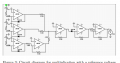#### WBahn

Joined Mar 31, 2012
27,896
Thanks. However, how would you explain the difference in behaviour I described between addition/subtraction, multiplication and division? Multiplication and division are nearly exactly the same voltage, yet they pose totally different results.
Thanks. However, how would you explain the difference in behaviour I described between addition/subtraction, multiplication and division? Multiplication and division are nearly exactly the same voltage, yet they pose totally different results.
For multiplication and division you are essentially taking the logarithm of the input, adding the logs, and then taking the exponential of the sum. The diodes are performing the log and antilog functions. Just as when you use logarithms by hand, the compression of values makes them much more susceptible to errors in offsets and gains, not to mention parametric mismatch between components, which is going to be pretty significant in three off-the-shelf diodes.

#### WBahn

Joined Mar 31, 2012
27,896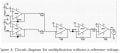I was about to show how slight variations in component parameters can lead to significant deviations in circuit behavior by assuming everything was perfect except one of the resistors that was off by 1%, but is starting I noticed something far more fundamental.

Even assuming that we are only talking about non-negative input values, when you multiply X by Y you can get a value that is less than X if Y is less than 1.

Yet, in this circuit, there is nothing about V=1 V (or any other non-negative voltage) that would cause such a fundamental change in behavior.

As long as V1 and V2 are both greater than 0 V, they result in a current that gets added together and, here's the important thing, that is greater (in magnitude) than either current alone. This means that you are adding logarithms together that are both positive and, when you take the antilog, you will get a result that is larger than either value by itself.

So there is a fundamental scaling/offset issue with the basic design of this circuit.